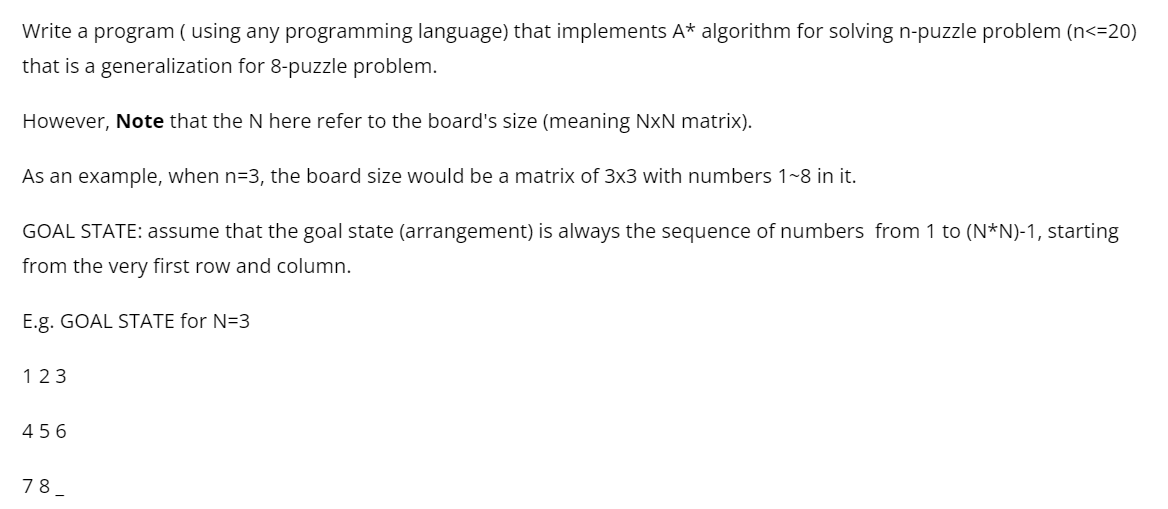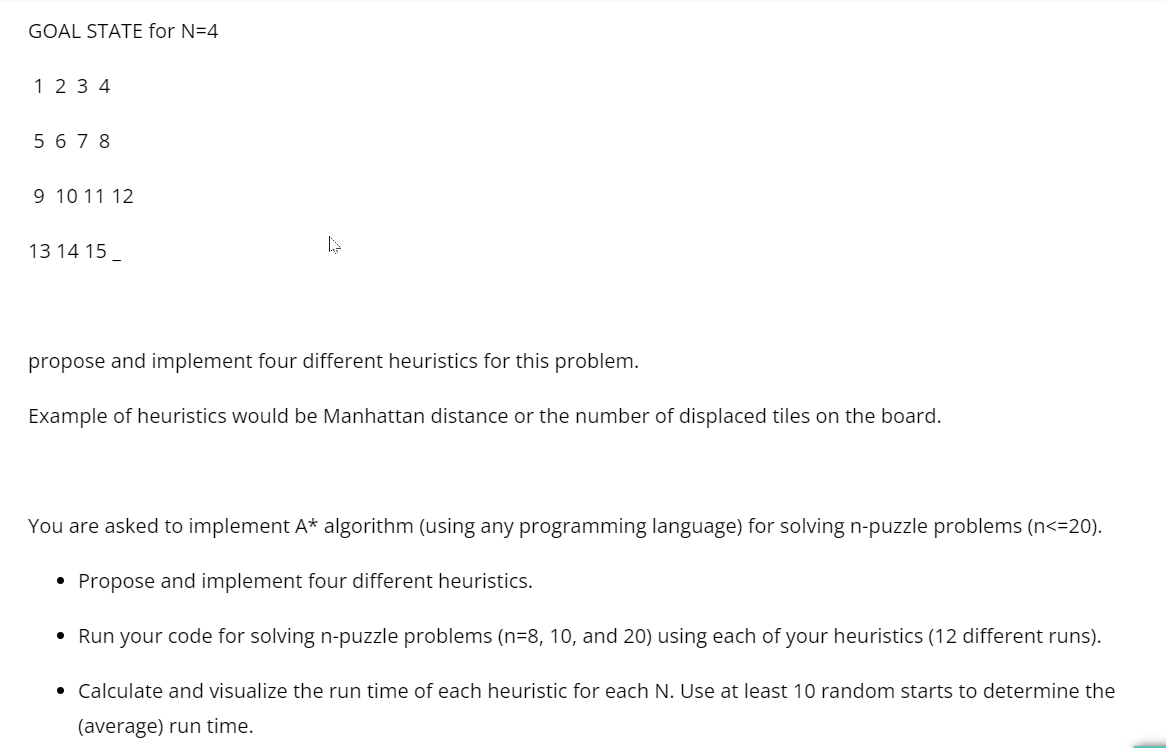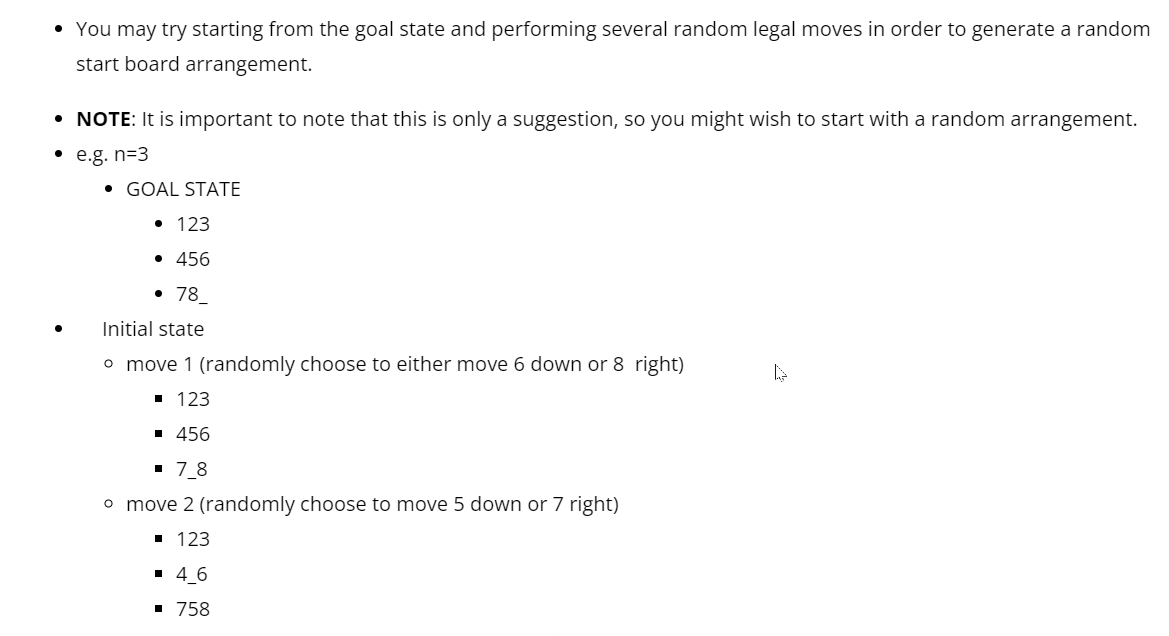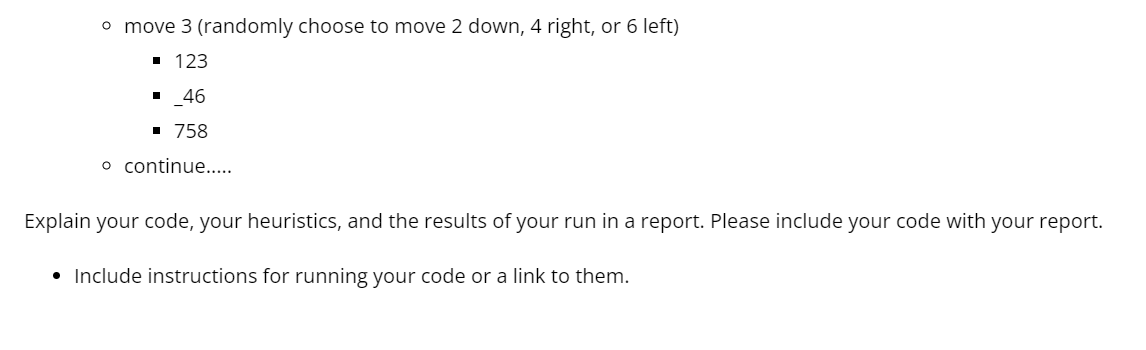# Question Write a program ( using any programming language) that implements $$A^{*}$$ algorithm for solving $$n$$-puzzle problem ( $$<=20$$ ) that is a generalization for 8 -puzzle problem. However, Note that the $$N$$ here refer to the board's size (meaning NxN matrix). As an example, when $$n=3$$, the board size would be a matrix of $$3 \times 3$$ with numbers $$1 \sim 8$$ in it. GOAL STATE: assume that the goal state (arrangement) is always the sequence of numbers from 1 to ( $$\left.N^{*} N\right)-1$$, starting from the very first row and column. E.g. GOAL STATE for $$\mathrm{N}=3$$ 123 456 78 propose and implement four different heuristics for this problem. Example of heuristics would be Manhattan distance or the number of displaced tiles on the board. You are asked to implement $$A^{*}$$ algorithm (using any programming language) for solving n-puzzle problems ( $$<=20$$ ). - Propose and implement four different heuristics. - Run your code for solving $$n$$-puzzle problems ( $$n=8,10$$, and 20) using each of your heuristics (12 different runs). - Calculate and visualize the run time of each heuristic for each N. Use at least 10 random starts to determine the (average) run time. - You may try starting from the goal state and performing several random legal moves in order to generate a random start board arrangement. - NOTE: It is important to note that this is only a suggestion, so you might wish to start with a random arrangement. - e.g. $$\mathrm{n}=3$$ - GOAL STATE - 123 - 456 - 78_ - Initial state - move 1 (randomly choose to either move 6 down or 8 right) - 123 - 456 - 7_8 - move 2 (randomly choose to move 5 down or 7 right) - 123 - 4_6 - 758 - move 3 (randomly choose to move 2 down, 4 right, or 6 left) - 123 - 46 758 - continue.... Explain your code, your heuristics, and the results of your run in a report. Please include your code with your report. - Include instructions for running your code or a link to them.U08TYM The Asker · Computer ScienceTranscribed Image Text: Write a program ( using any programming language) that implements $$A^{*}$$ algorithm for solving $$n$$-puzzle problem ( $$<=20$$ ) that is a generalization for 8 -puzzle problem. However, Note that the $$N$$ here refer to the board's size (meaning NxN matrix). As an example, when $$n=3$$, the board size would be a matrix of $$3 \times 3$$ with numbers $$1 \sim 8$$ in it. GOAL STATE: assume that the goal state (arrangement) is always the sequence of numbers from 1 to ( $$\left.N^{*} N\right)-1$$, starting from the very first row and column. E.g. GOAL STATE for $$\mathrm{N}=3$$ 123 456 78 propose and implement four different heuristics for this problem. Example of heuristics would be Manhattan distance or the number of displaced tiles on the board. You are asked to implement $$A^{*}$$ algorithm (using any programming language) for solving n-puzzle problems ( $$<=20$$ ). - Propose and implement four different heuristics. - Run your code for solving $$n$$-puzzle problems ( $$n=8,10$$, and 20) using each of your heuristics (12 different runs). - Calculate and visualize the run time of each heuristic for each N. Use at least 10 random starts to determine the (average) run time. - You may try starting from the goal state and performing several random legal moves in order to generate a random start board arrangement. - NOTE: It is important to note that this is only a suggestion, so you might wish to start with a random arrangement. - e.g. $$\mathrm{n}=3$$ - GOAL STATE - 123 - 456 - 78_ - Initial state - move 1 (randomly choose to either move 6 down or 8 right) - 123 - 456 - 7_8 - move 2 (randomly choose to move 5 down or 7 right) - 123 - 4_6 - 758 - move 3 (randomly choose to move 2 down, 4 right, or 6 left) - 123 - 46 758 - continue.... Explain your code, your heuristics, and the results of your run in a report. Please include your code with your report. - Include instructions for running your code or a link to them.
More
Transcribed Image Text: Write a program ( using any programming language) that implements $$A^{*}$$ algorithm for solving $$n$$-puzzle problem ( $$<=20$$ ) that is a generalization for 8 -puzzle problem. However, Note that the $$N$$ here refer to the board's size (meaning NxN matrix). As an example, when $$n=3$$, the board size would be a matrix of $$3 \times 3$$ with numbers $$1 \sim 8$$ in it. GOAL STATE: assume that the goal state (arrangement) is always the sequence of numbers from 1 to ( $$\left.N^{*} N\right)-1$$, starting from the very first row and column. E.g. GOAL STATE for $$\mathrm{N}=3$$ 123 456 78 propose and implement four different heuristics for this problem. Example of heuristics would be Manhattan distance or the number of displaced tiles on the board. You are asked to implement $$A^{*}$$ algorithm (using any programming language) for solving n-puzzle problems ( $$<=20$$ ). - Propose and implement four different heuristics. - Run your code for solving $$n$$-puzzle problems ( $$n=8,10$$, and 20) using each of your heuristics (12 different runs). - Calculate and visualize the run time of each heuristic for each N. Use at least 10 random starts to determine the (average) run time. - You may try starting from the goal state and performing several random legal moves in order to generate a random start board arrangement. - NOTE: It is important to note that this is only a suggestion, so you might wish to start with a random arrangement. - e.g. $$\mathrm{n}=3$$ - GOAL STATE - 123 - 456 - 78_ - Initial state - move 1 (randomly choose to either move 6 down or 8 right) - 123 - 456 - 7_8 - move 2 (randomly choose to move 5 down or 7 right) - 123 - 4_6 - 758 - move 3 (randomly choose to move 2 down, 4 right, or 6 left) - 123 - 46 758 - continue.... Explain your code, your heuristics, and the results of your run in a report. Please include your code with your report. - Include instructions for running your code or a link to them.